Math Olympiad Test: Triangles - 2

# Math Olympiad Test: Triangles - 2

Test Description

## 15 Questions MCQ Test Olympiad Preparation for Class 10 | Math Olympiad Test: Triangles - 2

Math Olympiad Test: Triangles - 2 for Class 10 2023 is part of Olympiad Preparation for Class 10 preparation. The Math Olympiad Test: Triangles - 2 questions and answers have been prepared according to the Class 10 exam syllabus.The Math Olympiad Test: Triangles - 2 MCQs are made for Class 10 2023 Exam. Find important definitions, questions, notes, meanings, examples, exercises, MCQs and online tests for Math Olympiad Test: Triangles - 2 below.
 1 Crore+ students have signed up on EduRev. Have you?
Math Olympiad Test: Triangles - 2 - Question 1

### In an isosceles triangle AB = AC = 25 cm BC = 14 cm. What is the length of altitude from A on BC?

Detailed Solution for Math Olympiad Test: Triangles - 2 - Question 1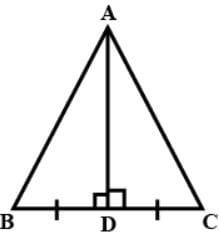Here altitude from A to BC is AD, we know in isosceles altitude on non-equal sides also median,
so, BD = CD = BC​/2 = 14​/2 = 7cm
Now, we applying Pythagoras theorem in △ABD,

Math Olympiad Test: Triangles - 2 - Question 2

### The area of two equilateral triangle are in the ratio 196 : 169. What is the ratio between their perimeters?

Detailed Solution for Math Olympiad Test: Triangles - 2 - Question 2

Ratio of perimeters = ratio of sides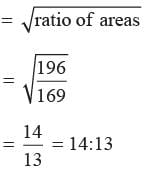Math Olympiad Test: Triangles - 2 - Question 3

### D and E are points on the sides AB and AC respectively of a DABC such that DE || BC find value of x if AD = (7x - 4) cm, AE = (5x - 2) cm, DB = (3x - 4) cm, EC = 3x cm

Detailed Solution for Math Olympiad Test: Triangles - 2 - Question 3

∵ DE ║ BE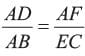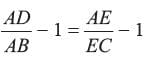⇒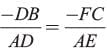adjusting this expression, we have
⇒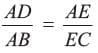⇒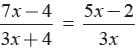⇒ 21x2 - 12x = 15x2 − 6x − 8 + 20 x
⇒ 6x2 − 26x + 8 = 0
⇒ 6x2 −24x − 2x + 8 = 0
⇒ 6x(x − 4) − 2(x − 4) = 0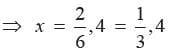When,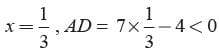∴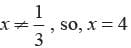Math Olympiad Test: Triangles - 2 - Question 4

In the given figure in DPQR, ST || QR, so that PS = (7x - 4) cm, PT = (5x - 2) cm, QS = (3x + 4) cm, RT = 3x cm.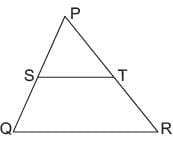What is the value of x?

Detailed Solution for Math Olympiad Test: Triangles - 2 - Question 4

In ΔPQR and ΔPST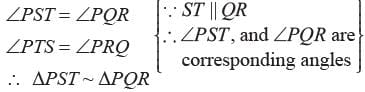⇒ According to Thales’ theorem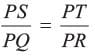Subtracting unity from both sides, we have,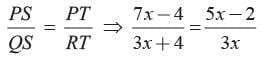⇒ 21x- 12x = 15x2 - 6x + 20x - 8
⇒ 6x2 - 26x + 8 = 0
⇒ 3x2 - 13x + 4 = 0
⇒ 3x2 - 12x - x + 4 = 0
⇒ 3x(x - 4) - 1(x - 4) = 0
⇒ (x - 4) (3x - 1) = 0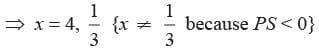∴ x = 4

Math Olympiad Test: Triangles - 2 - Question 5

A ladder 15 m long reaches a window which is 9 m above the ground on one side of a street. Keeping its foot at the same point, the ladder is turned to the other side of the street to reach a window 12 m high. What is the width of the street?

Detailed Solution for Math Olympiad Test: Triangles - 2 - Question 5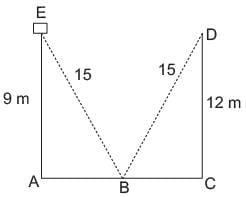In ΔABE,
AE2 + AB2 = BE2
⇒ 92 + AB2 = 152
⇒ AB2 = 225 - 81
= 144
⇒ AB = 12 m
Similarly,
In DBCD
⇒ BC2 = BD2 - DC2 = 152 - 122 = 92
BC = 9m
∴ width of street = (AB + BC) = 12 + 9 = 21 m

Math Olympiad Test: Triangles - 2 - Question 6

The corresponding altitude of two similar triangle are 6 cm and 9 cm respectively. What is ratio of their areas?

Detailed Solution for Math Olympiad Test: Triangles - 2 - Question 6

Ratio of areas = (ratio of altitudes)2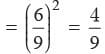Math Olympiad Test: Triangles - 2 - Question 7

In the given figure, ΔODC ~ ΔOBA, ∠BOC = 115°, ∠CDO = 70°. What is the value of ∠OAB?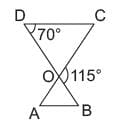Detailed Solution for Math Olympiad Test: Triangles - 2 - Question 7

∠ CDO + ∠DCO = 115°
⇒ ∠DCO = 115° - 70° = 45°
∠OAB = 45° (∴ DODC ~ DOBA)

Math Olympiad Test: Triangles - 2 - Question 8

A tree is broken of 6 m from the ground and its top touches the ground at a distance of 8 m from the base of the tree. What is the original height of the tree?

Detailed Solution for Math Olympiad Test: Triangles - 2 - Question 8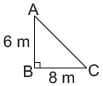A is the point of cleavage C is the point where top of three touches the ground
In ΔABC
AB2 + BC2 = AC2 (Pythagoras’ Theorem)
62 + 82 = AC2
= AC = 10 m
∴ Total (original) height = 10 m + 6 m = 16 m

Math Olympiad Test: Triangles - 2 - Question 9

An exterior angle of a triangle measures 110° and its interior opposite angles are in the ratio 2 : 3. Which is the largest angle of the triangle?

Detailed Solution for Math Olympiad Test: Triangles - 2 - Question 9

Let the angles be 2x and 3x respectively
∴ 2x + 3x = 110°
⇒ 5x = 110°
⇒ x = 22°
∴ Angles are 44°, 66°, and 70°

Math Olympiad Test: Triangles - 2 - Question 10

What is the perimeter of a rhombus the length of whose diagonal are 16 cm and 30 cm?

Detailed Solution for Math Olympiad Test: Triangles - 2 - Question 10

Let the side of rhombus be x cm
Now, we know that the diagonals of rhombus bisect each other at right angles.
∴ By using Pythagoras’ Theorem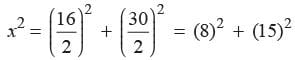= 64 + 225 = 289
⇒ x = 17 cm
∴ Perimeter =  4 × x = 4 × 17 = 68 cm.

Math Olympiad Test: Triangles - 2 - Question 11

In the given figure BC is parallel to DE. Area of DABC is 25 cm2. Area of trapezium BCED is 24 cm2, if DE = 14 cm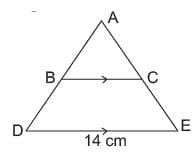What is the length of BC?

Detailed Solution for Math Olympiad Test: Triangles - 2 - Question 11

∵  BC ║ DE
∴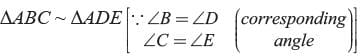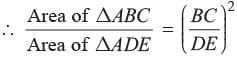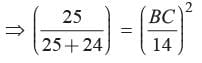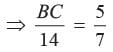⇒ BC = 10 cm

Math Olympiad Test: Triangles - 2 - Question 12

A man goes 10 m due south and then 24 m due west. How far is he from the starting point?

Detailed Solution for Math Olympiad Test: Triangles - 2 - Question 12

In ΔABC
AC2 = AB2 + BC2 (Pythagoras’ theorem)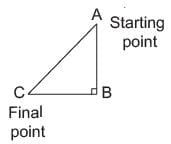⇒ AC =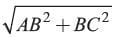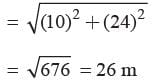Math Olympiad Test: Triangles - 2 - Question 13

A vertical pole 12 m long casts a shadow of 8 m long on the ground. At the same time, a tower casts the shadow 40 m long on the ground. What is the height of the tower?

Detailed Solution for Math Olympiad Test: Triangles - 2 - Question 13

Both Ds are right angled, and also the elevation of coming sunlight will be same.
∴ All the angles of the ΔABC and ΔPQR will be correspondingly identical.
∴ ΔABC ~ ΔPQR
⇒ AB/PQ = BC/QR
⇒ 12/PQ = 8/40
⇒ PQ = 12 × 5 = 60m

Math Olympiad Test: Triangles - 2 - Question 14

In an isosceles triangle ΔABC if AB = AC = 13 cm and altitude from A on BC is 5 cm. What is the length of BC?

Detailed Solution for Math Olympiad Test: Triangles - 2 - Question 14

In ΔABD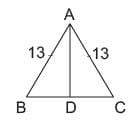⇒ BD =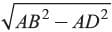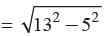= 12 cm
∵ D bisects BC
∴ BC = 2 × BD
= 2 × 12 = 24 cm

Math Olympiad Test: Triangles - 2 - Question 15

The Perimeter of two similar triangles are 25 cm and 15 cm respectively. If one side of first triangle is 9 cm. Then what is the corresponding side of the second circle?

Detailed Solution for Math Olympiad Test: Triangles - 2 - Question 15

We know that if, ΔABC ~ ΔPQR, then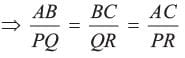or, Applying componendo and dividendo, it can be clearly seen that,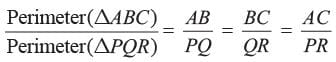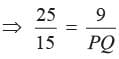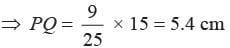## Olympiad Preparation for Class 10

11 videos|36 docs|201 tests
 Use Code STAYHOME200 and get INR 200 additional OFF Use Coupon Code